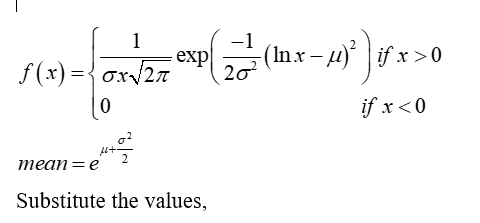# The prices of stocks or other financial instruments are often modeled with a lognormal distribution. An investor is considering purchasing stock in one of two companies, A or B. The price of a share of stock today is \$1 for both companies. For company A, the value of the stock one year from now is modeled as lognormal with parameters μ = 0.05 and σ = 0.1. For company B, the value of the stock one year from now is modeled as lognormal with parameters μ = 0.02 and σ = 0.2. a) Find the mean of the price of one share of company A one year from now. b) Find the probability that the price of one share of company A one year from now will be greater than \$1.20. c) Find the mean of the price of one share of company B one year from now. d) Find the probability that the price of one share of company B one year from now will be greater than \$1.20.

Question
1 views

The prices of stocks or other financial instruments are often modeled with a lognormal distribution. An investor is considering purchasing stock in one of two companies, A or B. The price of a share of stock today is \$1 for both companies. For company A, the value of the stock one year from now is modeled as lognormal with parameters μ = 0.05 and σ = 0.1. For company B, the value of the stock one year from now is modeled as lognormal with parameters μ = 0.02 and σ = 0.2. a) Find the mean of the price of one share of company A one year from now. b) Find the probability that the price of one share of company A one year from now will be greater than \$1.20. c) Find the mean of the price of one share of company B one year from now. d) Find the probability that the price of one share of company B one year from now will be greater than \$1.20.

check_circle

Step 1

Given that,

For company A, the value of the stock one year from now is modeled as lognormal with parameters μ = 0.05 and σ = 0.1.

For company B, the value of the stock one year from now is modeled as lognormal with parameters μ = 0.02 and σ = 0.2.

Step 2

a)

The random variable X is defined as the price of a share of company A

Let Y is defined as the price of a share of company B

Lognormal distribution:

The probability density function of a lognormal random variable with parameters µ and σ,

Step 3...

### Want to see the full answer?

See Solution

#### Want to see this answer and more?

Solutions are written by subject experts who are available 24/7. Questions are typically answered within 1 hour.*

See Solution
*Response times may vary by subject and question.
Tagged in